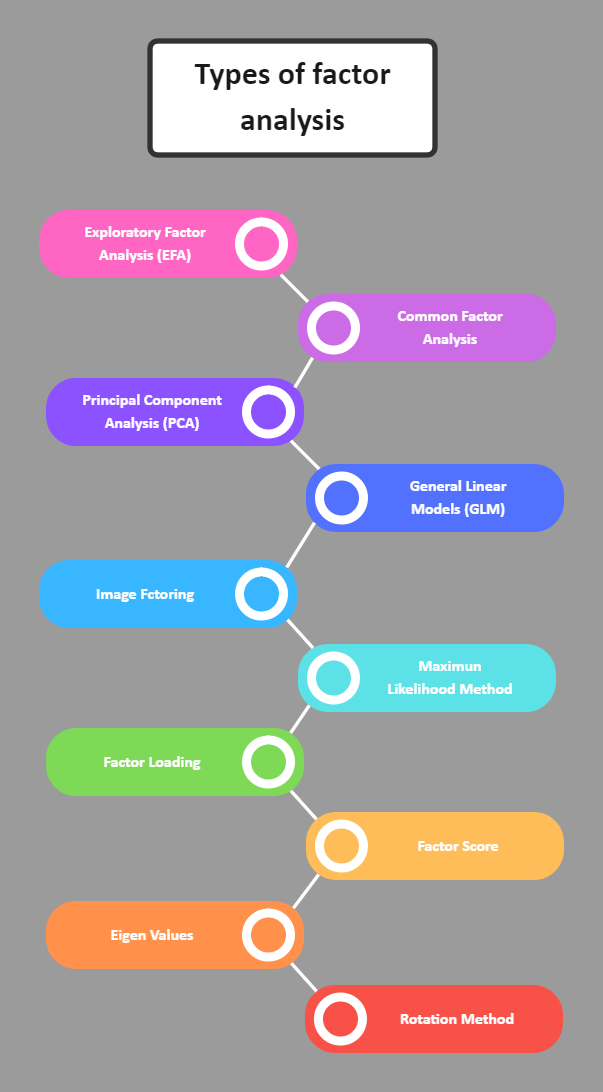Template Community / Types of Factor Analysis

# Types of Factor AnalysisCommunity Helper
Published on 2022-08-24
Edit OnlineWe use various types of factor analysis in data set factor analysis. Factor analysis is a mathematical technique for reducing a large number to a smaller number. Furthermore, we will discuss it and its various aspects on this topic. It refers to a method for converting a large variable into a smaller variable factor. Furthermore, this technique extracts the maximum ordinary variance from all variables and assigns it to a single score. Moreover, it is a General Linear Model (GLM) component. It incorporates theories that include no multicollinearity, linear relationship, true correlation, and relevant variables in analyzing factors and variables, as illustrated in this Types of Factor Analysis diagram.
Tag